Click to Chat

1800-1023-196

+91-120-4616500

CART 0

• 0

MY CART (5)

Use Coupon: CART20 and get 20% off on all online Study Material

ITEM
DETAILS
MRP
DISCOUNT
FINAL PRICE
Total Price: Rs.

There are no items in this cart.
Continue Shopping• Complete Physics Course - Class 11
• OFFERED PRICE: Rs. 2,968
• View Details

Gravitational Potential Energy

Table of Content

Gravitational Potential Energy of a System

Gravitational Potential

Gravitational Potential due to a Shell

Gravitational Potential (V) due to a Uniform Solid Sphere

Relation between Gravitational Potential and the Gravitational Field Strength

Escape Velocity

Binding Energy

Gravitational Self Energy of a Uniform Sphere

Related Resources

When two or more bodies interact with each other, some work has to be done in assembling them together in their respective places.

Total work done in assembling bodies together, in their respective places, is called gravitational potential energy of the system.

Potential energy of a system of two masses is defined as the amount of work done in bringing these two masses from infinity to their respective places.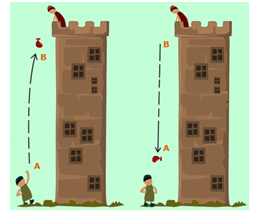In the right side figure, as the bag moves upwards, kinetic energy decreases, and gravitational energy increases. At the highest point (B) Kinetic energy is zero, and Gravitational potential energy is highest.

As the bag did not get to, it start falling from point B downwards due to gravity. It starts falling slowly (kinetic energy is low) and then speeds up downwards.

At point A, bag is at full speed and kinetic energy is highest, whiles gravitational energy is nearly lost.

When the bag of gold coins hits the ground, kinetic energy is converted into heat and sound by the impact.

It is worth noting that the higher the gravitational energy of an object moving downwards, the lower the kinetic energy, and the lower the kinetic energy of an object moving upwards, the higher its gravitational energy.

Gravitational Potential Energy of a System

Change in gravitational potential energy of a system is defined as the -ve of the work done by the gravitational force as the configuration of the system is changed.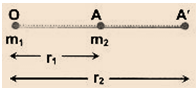Uf - Ui = Wgr

Change in gravitational potential energy of two point masses m1 and m2 as their separation is changed from r1 to r2 is given by

U(r2) - U(r1) = Gm1m2 [1/r1 - 1/r2]

If, at infinite separation, gravitational potential energy is assumed to be zero, then the gravitational potential energy of the above two point mass system at separation r,The below figure shows that the energy of the dart/gun system is initially present in the form of the elastic potential energy (PEs) and gravitational potential energy (PEg). The springs of the dart gun are compressed which accounts for the elastic potential energy. Furthermore, the dart is initially elevated at a height of 1-meter above the ground which accounts for the gravitational potential energy. The presence of these two initial forms of energy are shown by the PEg and PEs bars of the bar chart. Once projected, the dart no longer has elastic potential energy since the springs of the dart are no longer compressed. However, the dart does have a large amount of kinetic energy (energy of motion) since it is now moving at a high speed as it leaves the dart. The dart also has gravitational potential energy since it is still elevated to some height above the ground. As the dart ascends towards its peak, it is continuously slowing down under the influence of the downward force of gravity. During this ascent, there is a transformation of the mechanical energy from the form of kinetic energy (energy of motion) to gravitational potential energy (the stored energy of vertical position). At the peak, there is only a small amount of kinetic energy (the dart still has a horizontal motion) and a large amount of gravitational potential energy (the dart is at its highest vertical position). Finally, as the dart descends to the ground, the force of gravity speeds it up. As it falls, there is an increase in kinetic energy (due to the gain in speed) and a decrease in gravitational potential energy (due to a loss in vertical position).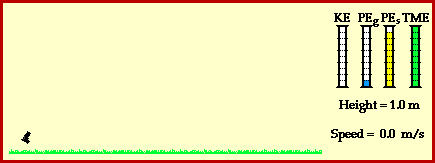Gravitational Potential

Gravitational field around a material body can be described not only by gravitational intensity vector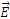but also by a scalar function, the gravitational potential V. The gravitational at any point may be defined as the potential energy per unit mass of a test mass placed at that point.

V = U/m  (where U is the gravitational potential energy of the test mass m).

Thus, if the reference point is taken at infinite distance, the potential at a point in the gravitational field is equal to amount of work done by the external agent per unit mass in bringing a test mass from infinite distance to that point. The expression for the potential is given by

With the above definition, the gravitational potential due to a point mass M at a distance r from it is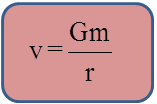Potential is a scalar quantity. Therefore, at a point in the gravitational field of a number of material particles, the resultant potential is the arithmetic sum of the potentials due to all the particles at that point. If masses m1, m2, ......, mn are at distances r1, r2, r3, ......, rn then potential at the given point is

V = -G(m1/r1 + m2/r2 + m3/r3 +..........)

The field and the potential are related as, E = -dV/dr

Refer this video for better understanding about gravitational potential energy:-

Gravitational Potential due to a Shell

(i)  at a point outside the shell is: -GM/r (r>R)

(ii)  at a point on the surface of the shell is: -GM/R

(iii)  at a point inside the shell is: -GM/R

Gravitational Potential (V) due to a Uniform Solid Sphere

(i)  Outside of the sphere at a distance r form the center, V = -GM/r.

(ii)  Inside the sphere at a distance r from the center, V = -GM/R3 (R2/2 - r2/6)

Relation between Gravitational Potential and the Gravitational Field Strength

Gravitational field strength at a point is given by

E = GM/r2

We can also describe the gravitational field of a body by a scalar function called the potential. Let us begin with a test particle of mass m0 at an infinite separation from the body and move the test particle toward the body until their separation is r, where the potential energy is U(r). Now the gravitational potential V at that point is defined as V(r) = U(r)/m0.

As we can calculate the radial component of gravitational forcefrom U(r) according to the relation

F = -dU/dr   =>  m0E = -m0 dv(r)/dr

Hence, we can find the radial component of the gravitational field intensity from V(r)

According to the relation, E = -dV/dr.Escape Velocity

Escape velocity on the surface of earth is the minimum velocity given to a body to make it free from the gravitational field, i.e. it can reach an infinite distance from the earth.

Let ve be the escape velocity of the body on the surface of earth and the mass of the body to be projected be m. Now, conserving energy at the surface of the earth and infinity,

1/2 mve2 - GMm/R = 0 => ve = √2GM/R.Binding Energy

Binding energy of a system of two bodies is the amount of minimum energy needed to separate the bodies to a large distance.

If two particles of masses m1 and m2 are separated by a distance r, then the gravitational potential energy of the system is given by

U = Gm1m2/r

Let T amount of energy is supplied to the system to separate the bodies by a large distance. When the bodies are separated by a large distance, gravitational potential energy of the system is zero. For minimum T, conserving energy for initial and final positions,

T + U = 0

=>    T - Gm1m2/r = 0 or T = Gm1m2/r

Hence, binding energy of a system of two particles separated by a distance r is equal to

T = Gm1m2/r, where m1 and m2 are the masses of the particles.

Gravitational Self Energy of a Uniform SphereConsider a sphere of radius R and mass M uniformly distributed. Consider a stage of formation at which the radius of the spherical core is r. Its mass will be 4/3 πr3ρ at that time, where r is the density of the sphere.

Let an additional mass be added so that the radius of the core be increased by dr in the form of a spherical layer over the core. The mass of this layer will be 4πr2dr. ρ

The mutual gravitational potential energy of the above mass and the spherical core of radius r,

dU = (-G(4/3πr3ρ)(4πr2dr.ρ))/r = 16/3 π2ρ2Gr4dr

Hence, total energy evolved in the formation of the spherical body of radius R i.e., self energy.Gravitational potential at any point is a scalar quantity.

Its unit, in C.G.S. system, is erg g-1 and in S.I. it is J kg-1.

With an increase in ‘r’, it becomes less negative i.e., it increases as we move away from the source of gravitational fleld.

Maximum value of gravitational potential is zero and this happens only at infinite distance from the source.

?

Problem 1:-

What is the gravitational Potential energy of the Moon-Earth system, relative to the potential energy at infinite separation?

Solution:-

M = Mass of Earth = 5.98 × 1024 kg

m = mass of Moon = 7.36 = 1022 k

d = mean separation between Earth and moon = 3.82 × 108 m.

So, gravitational potential energy of the Moon-Earth system (U)

= -GMm/d

Put all values U = -7.68 × 1028 J

______________________________________________________________________________________

Problem 2:-

A projectile is fired vertically from the Earth's surface with an initial velocity of 10 km/s. Neglecting atmospheric retardation, how far above the surface of the Earth would it go? Take the earth's radius as 6400 km.

?Solution:-

Let the projectile go up to a height h. Then the law of conservation of mechanical energy gives

1/2 mv2 - GMm/R = -GMm/R+h

h = v2R2/2GM-v2R

= v2R2/2gR2-v2R

where g is the acceleration due to gravity on the surface of the Earth.

= ((104)2 (6.4×106)2)/(2(9.8)(6.4×106)2-(104)2 (6.4×106))

_____________________________________________________________________________________

Problem 3:-Two spherical bodies of masses 2M and M and of radii 3R and R, respectively, are held at a distance 16R from each other in free space. When they are released, they start approaching each other due to the gravitational force of attraction. Then, find:

(a)    the ratio of their acceleration during their motion.

(b)    their velocities at the time of impact.

?Solution:-

(a) Due to the mutual attraction, the masses attract each other.

If the acceleration are a1 and a2, the net external force on the system = 0

=>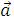cm = 0 => m1a1 - m2a2 = 0

or a1/a2 = m2/m1 = ½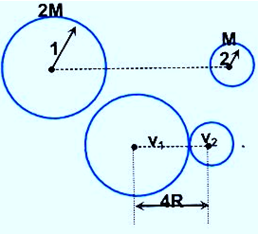(b) Taking both the bodies as a system, from conserving momentum of the system,

m1v1 - m2v2 = 0 => m1/m2 = v2/v1 = 2

Now, conserving the total mechanical energy, we have

1/2 (2M) V12 + 1/2MV22 - G(2m)M/4R = - G(2m)M/16R and solving it we get.

v1 = √GM/8R and v2 = 2√GM/8RNote:

The velocities and acceleration are w.r.t. the inertial reference frame (i.e. the center of mass of the system).

Problem 4:-

At a point above the surface of earth, the gravitational potential is  -5.12 × 107J/kg and the acceleration due to gravity is 6.4 m/s2. Assuming the mean radius of the earth to be 6400 km, calculate the height of this point above the earth's surface.

?Solution:-

Let r be the distance of the given point from the centre of the earth. Then,

Gravitational potential = -GM/r = -5.12 × 107 J/kg               ...... (1)

and acceleration due to gravity,

g = GM/r2 = 6.4 m/s2                                               ...... (2)

Dividing (1) by (2), we get

r = 5.12*107/6.4 = 8 × 106 m = 8000km

Thus, height of the point from earth's surface = r - R = 8000 - 6400 = 1600 km

______________________________________________________________________________________

Problem 5:-

The mass of Jupiter is 318 times that of earth, and its radius is 11.2 times the earth's radius. Estimate the escape velocity of a body from Jupiter's surface. [Given: The escape velocity from the earth's surface is 11.2 km/s.]

?Solution:-

Hence, MJ = 318 Me; RJ = 11.2 Re; ve = 11.2 km/s

We know, vJ = √2GMj/Rj and ve = √2GMe/Re

so, vJ/ve = √(Mj/Me χ Re/Rj)

=> vJ = ve √(Mj/Me χ Re/Rj)

vJ = 11.2{318Me/Me χ Re/11.2Re}1/2  = 11.2(318/11.2)1/2 = 59.7 km/s

___________________________________________________________________________________

Problem 6:-

Find the escape speed from a point at a height of R/2 above the surface of earth. Assuming mass of earth as M and its radius as R.

?Solution:-

Conserving mechanical energy of a point mass m which is to escape, and earth system we have,

-GMm/(R+R/2) + 1/2mv2 = 0

=> v = √(4GM/3R)

________________________________________________________________________________________

Problem 7:-

The escape velocity of a body on the surface of the Earth is 11.2 km/s. A body is projected away with twice this speed. What is the speed of the body at infinity? Ignore the presence of other heavenly bodies.

?Solution:-

If v is the velocity of projected and v' is the velocity at infinity, then we have by energy conservation principle.

½ mv2 - GMm/R = 1/2mv'2 + 0

Here  v = 2ve

Thus, (1/2) . 4ve2 - GM/R = 1/2v'2

=> 2ve2 - GM/R = 1/2v'2

Now  ve = √2GM/R

=> 2ve2 - v2e/2 = 1/2v'2

or, v'2 = 3  ve2

or, v' = √3 ve = √3 × 11.2 km/s = 19.4 km/s.Question 1:-

A ball is thrown up vertically in air. As it rises there is an increase in its

(a) potential energy        (b) Kinetic energy     (c) acceleration    (d) None of them

Question 2:-

The value of ‘g’ (acceleration due to gravity) is zero at

(a) Pole     (b) Equator    (c) The height of 1000 mile from surface of earth    (d) The center of earth

Question 3:-

The escape velocity of earth is

(a)1.12 km/s      (b) 11.2 m/s     (c) 11.2 km/hr      (d) 11.2 km/s

Question 4:-

A satellite revolves very near to the earth surface. Its speed should be around

(a) 5 km/s       (b) 8 km/s      (c) 2 km/s      (d) 11 km/s

Question 5:-

A geostationary satellite revolves round the earth from

(a) East to West     (b) West to East     (c) North to South      (d) South to NorthQ.1
Q.2
Q.3
Q.4
Q.5

a

d

d

b

b

Related Resources

You might like to refer Satellites and Planetary motion.

For getting an idea of the type of questions asked, refer the  Previous Year Question Papers.### Course Features

• 101 Video Lectures
• Revision Notes
• Previous Year Papers
• Mind Map
• Study Planner
• NCERT Solutions
• Discussion Forum
• Test paper with Video Solution